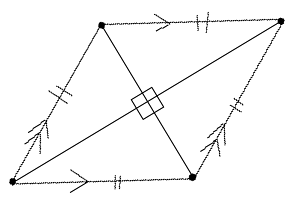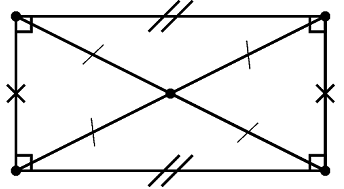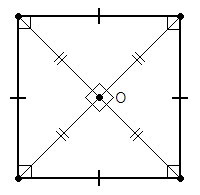# Special Parallelograms: Rhombus, Square & Rectangle

In Geometry, the shapes can be classified into two different categories, such as:

• Two – Dimensional Shapes
• Three – Dimensional Shapes.

Two – dimensional shapes or flat shapes are the shapes that contain only two dimensions, such as length and breadth. It does not have thickness. Some of the examples 0f 2D shapes are square, rectangle, triangle, and so on.

Three – dimensional shapes are also known as solids that contain three-dimensions such as length, breadth, and width. When flat shapes are rotated, the 3D shapes are obtained. The faces of the solids must be a 2D Shape. Some of the examples of the 3D shapes are a be, cuboid, cone, cylinder, and so on.

In this article, we are going to discuss the special parallelograms such as square, rectangle, and parallelogram in detail. Before talking about the special parallelograms, let us recall what a parallelogram is.

## What is Parallelogram?

A parallelogram is a quadrilateral with two pairs of parallel sides.  The opposite sides are parallel and equal in length. The opposite angles are equal in measure. The adjacent angles are supplementary and it has 2 diagonals that bisect each other. In this article, we will discuss some special parallelograms – rhombus, rectangle and square.

## Rhombus

A rhombus is a quadrilateral with all the four sides having equal lengths.### Properties of Rhombus

• In a rhombus, the opposite sides are parallel and equal. Hence, it is a parallelogram.
• However, all the 4 sides are equal in a rhombus.
• Opposite angles are equal.
• Diagonals of a rhombus intersect each other at right angles.
• Diagonals bisect each other.
• Diagonals bisect opposite vertex angles.
• Each of the diagonal divides the rhombus into 2 congruent triangles.

### Rhombus Formula

If  “a” is the side, “p and q” are the two diagonals of the rhombus, then the area and perimeter of the rhombus formulas are given as:

Area of Rhombus = pq/2 Square units.

Perimeter of Rhombus = 4a units

## Rectangle

A rectangle is a parallelogram with all the 4 angles having equal measure.### Properties of Rectangle

• Opposite sides are equal in length.
• Opposite sides are parallel.
• The interior angles measure 90 degrees each.
• Diagonals are equal in length.
• Diagonals bisect each other.
• Each of the diagonal bisects the rectangle into 2 congruent triangles.
• It has 2 lines of symmetry – a horizontal and a vertical.
• The mid-points of the 4 sides of a rectangle, when joined in order, form a rhombus.

### Rectangle Formula

If “l” and “b” are the length and breadth of the rectangles, then the area and the perimeter of a rectangle formula is given as:

Area of Rectangle = lb square units

The Perimeter of Rectangle = 2 (l+b) units.

## Video Lesson on Properties of rectangles## Square

A square is a rectangle with equal sides.### Properties of Square

• All the 4 sides are equal in length.
• Opposite sides are parallel.
• The interior angles measure 90 degrees each.
• Diagonals are equal in length.
• Diagonals bisect each other at right angles.
• Diagonals bisect opposite vertex angles.
• It is a highly symmetric figure with 4 lines of symmetry – a horizontal, a vertical and the 2 diagonals.
• Each of the diagonal bisects the square into 2 congruent triangles. In fact, both the pairs of congruent triangles are also congruent to one another.

### Square Formula

If “a” is the side of a square, then the area and the perimeter of a square formula is given as:

Area of Square = a2 square units

The Perimeter of square = 4a units.

### Problems on Special Parallelograms

Example 1: Find the area and the perimeter of a square whose side is 6 cm

Solution:

Given: Side, a = 6 cm

We know the formulas,

Area of Square = a2 square units

A = 6 × 6 = 36

Therefore, the area of a square is 36 cm2

The perimeter of a Square = 4a units

P = 4 × 6 = 24

Therefore, the perimeter of a square is 24 cm

Example 2:

Find the area and the perimeter of a rhombus whose diagonal measures are 3cm and 5cm and side measure is 4 cm.

Solution:

Given that,  side, a = 4 cm

Also, the diagonal measures are:

p = 3 cm and q = 5 cm

The formula to find the area and perimeter of a rhombus are:

Area of a rhombus, A = pq/2 square units

A = (3×5) / 2 = 15/2 = 7.5

Therefore, the area of a rhombus = 7.5 cm2

The perimeter of a rhombus, p = 4a square units

p = 4 × 4 = 16

Therefore, teh perimeter of a square = 16 cm

To learn more properties and formulas on different geometrical shapes, visit BYJU’S – The Learning App and download the app to learn with ease.

Quiz on Special Parallelograms: Rhombus, Square, Rectangle